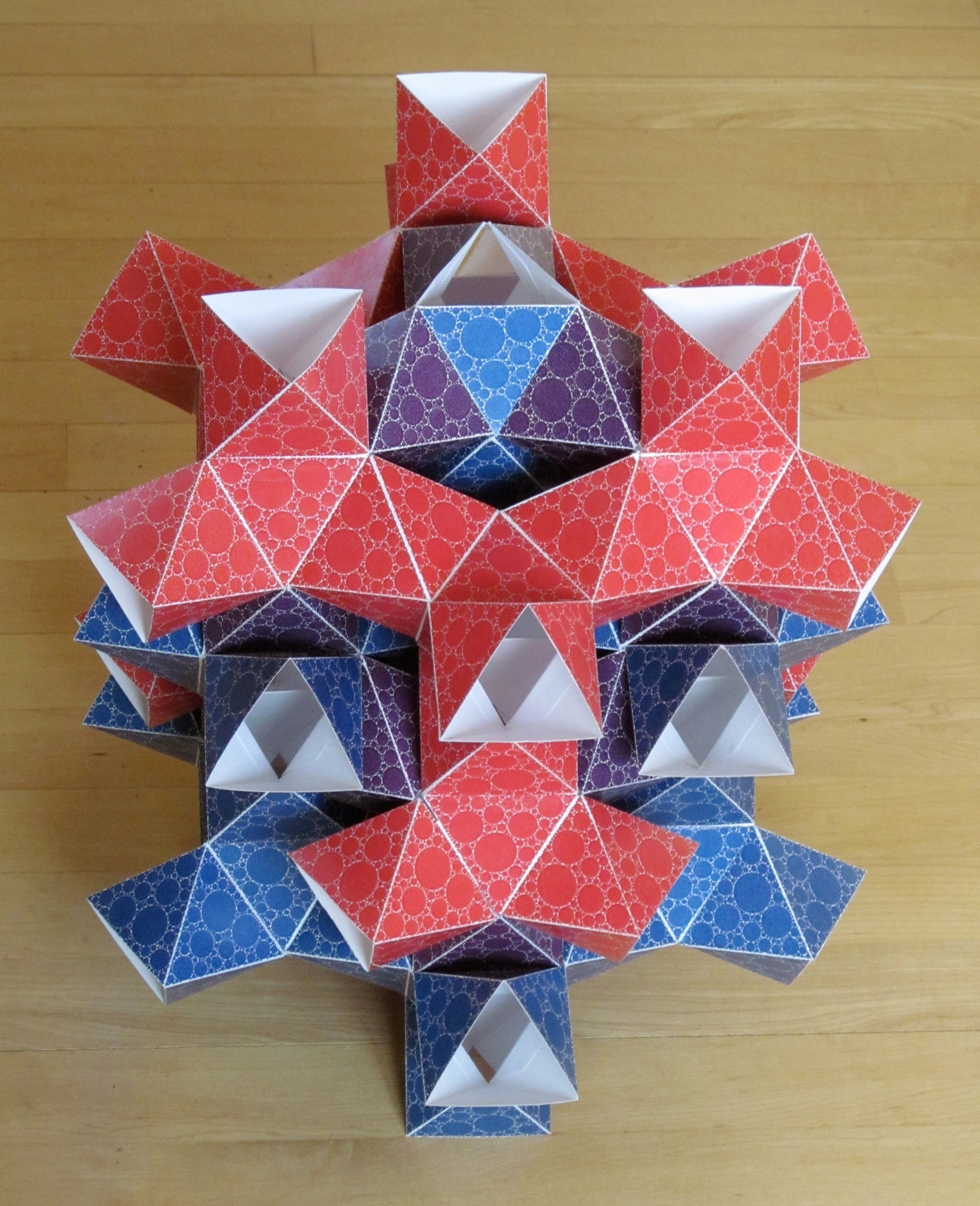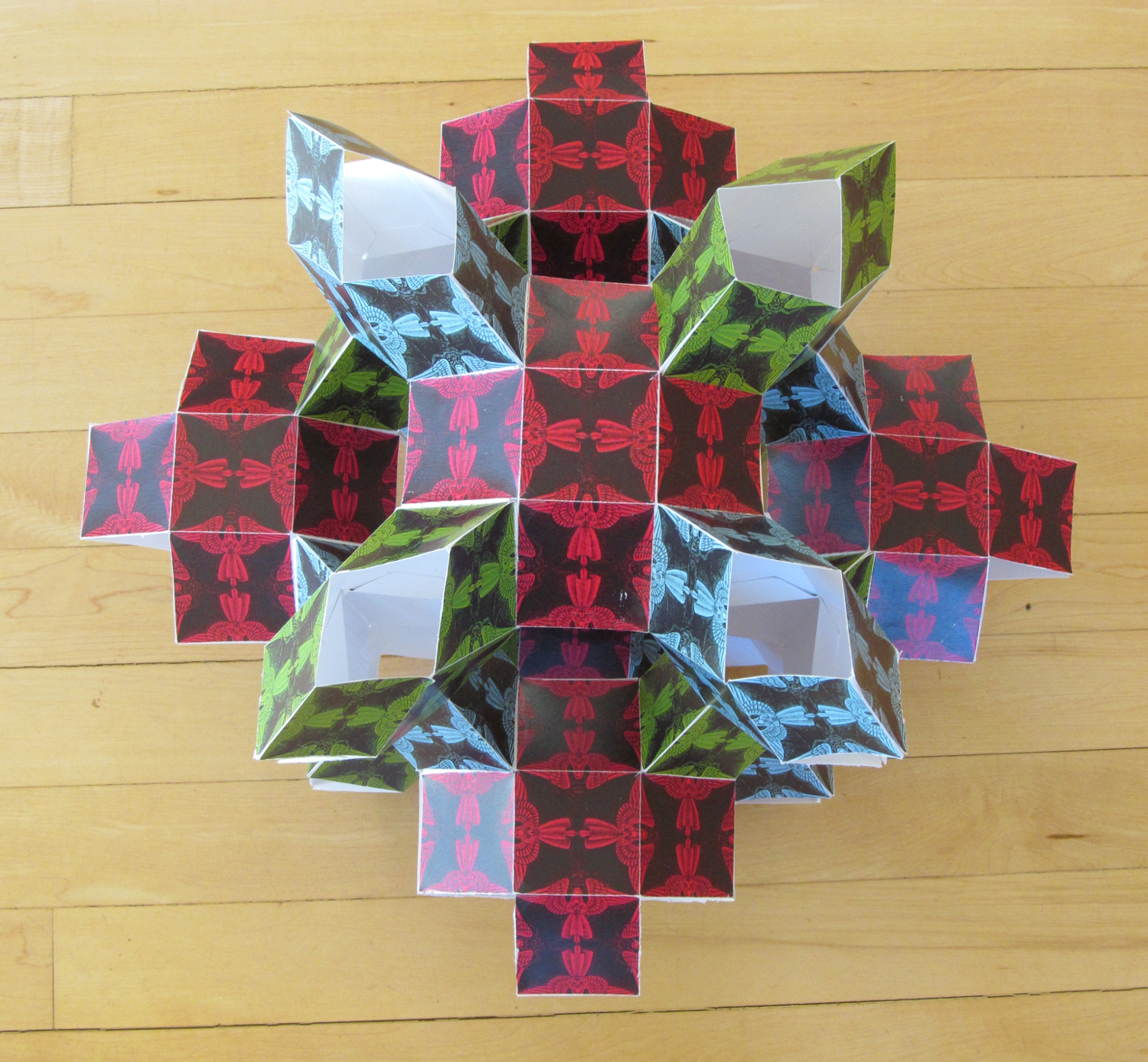# Doug Dunham

Professor of Computer Science
Department of Computer Science, University of Minnesota Duluth
Duluth, Minnesota, USA

The goal of my art is to create aesthetically pleasing repeating hyperbolic patterns. One way to do this is to place patterns on (connected) triply periodic polyhedra in Euclidean 3-space. These polyhedra can be considered to be hyperbolic since the sum of the angles around each vertex is greater than 360 degrees. The first polyhedron below is decorated with a fractal circle pattern. The second and third polyhedra are decorated with motifs insprired by M.C. Eshcer. There is a two-step connection between these latter two polyhedra and hyperbolic plane patterns: (1) they approximate triply periodic minimal surfaces, and (2) those surfaces have the hyperbolic plane as their universal covering surface.The {3,12} Polyhedron Decorated with a Fractal Circle Pattern
50 x 30 x 30 cm
Printed Cardboard
2015

This polyhedron is constructed by placing regular octahedra on all the faces of another such octahedron, so there will be 12 equilateral triangles about each vertex. Each of the triangular faces has been 90% filled by a fractal pattern of circles provided by John Shier. The polyhedron consists of red and blue "diamond lattice" polyhedra and purple octahedra that connect the red and blue polyhedra. Each of the red and blue polyhedra consists of octahedral "hubs" connected by octahedral "struts", each hub having 4 struts projecting from alternate faces. The red and blue polyhedra are in dual position with respect to each other - they form interlocking cages. Each purple connector has a red and a blue octahedron on opposite faces.Angels and Devils on a {4,5} Polyhedron
35 x 35 x 35 cm
Printed Cardboard
2013

The Dutch artist M.C. Escher created "Angels and Devils" patterns in the Euclidean plane, on the sphere, and in the hyperbolic plane ("Circle Limit IV"). These patterns were based respectively on the {4,4} (square) plane tessellation, on the {4,3} ("cubic") spherical tessellation, and on the {6,4} hyperbolic tessellation. So Escher didn't use the {4,5} tessellation. The {4,5} polyhedron above fills this gap. It is composed of modules consisting of 10 squares: two opposite faces of a cube and 4 equilateral triangular prisms each with a lateral side on one of the 4 remaining faces of the cube. It has the same topology of Schoen's IWP triply periodic minimal surface. It also exhibits 3-color symmetry.Butterflies on a {3,8} Polyhedron
37 x 37 x 37 cm
Printed Cardboard
2013

Unlike regular plane or spherical tessellations, the number of polygon sides and the number meeting at each vertex do not determine a triply periodic polyhedron. This polyhedron has the same Schlafli symbol {3,8} as the "diamond lattice" polyhedron described in the first figure above, but it is a different polyhedron. It consists of snub cubes of alternating chirality positioned in a cubic lattice connected by their (non-existing) square faces. Thus a "left" snub cube is attached to a neighborinig "right" snub cube by a common square. Each of the triangles is filled with butterflies of 3 colors, red, blue, and yellow. This pattern was inspired by M.C. Escher's Regular Division Drawing # 70. It has 3-color symmetry.